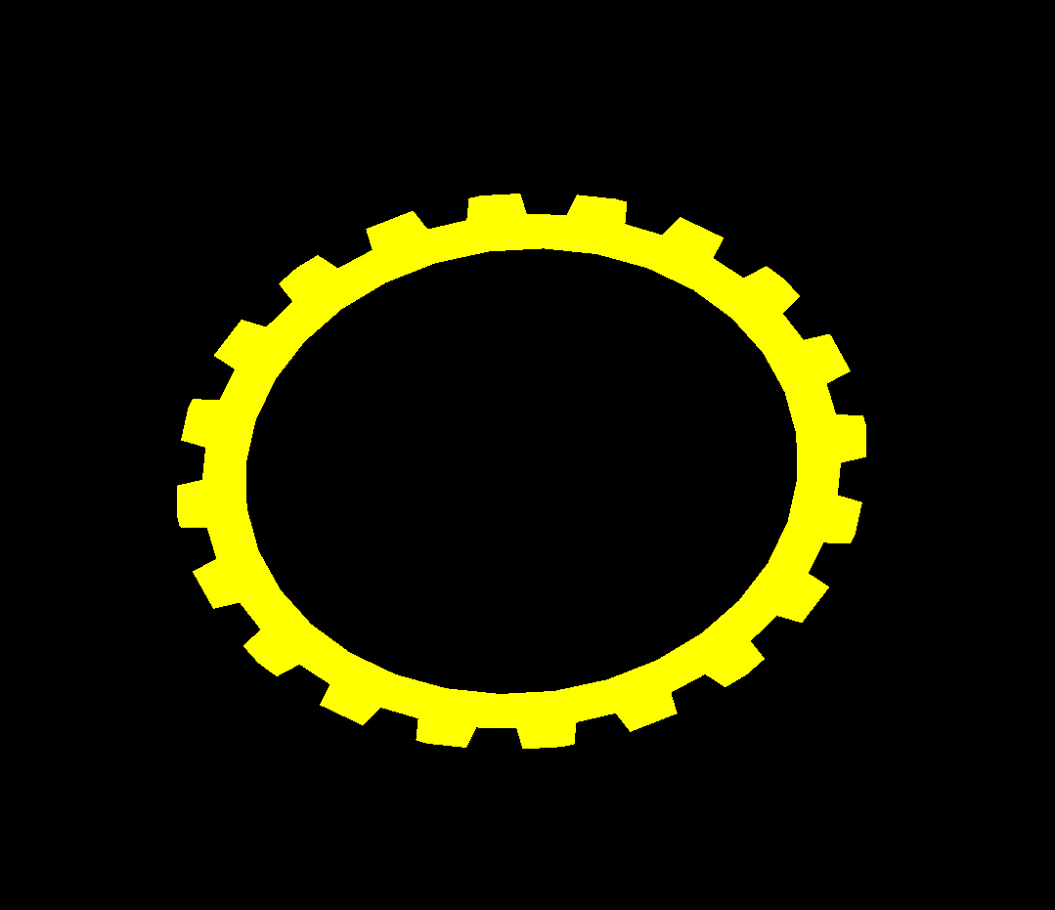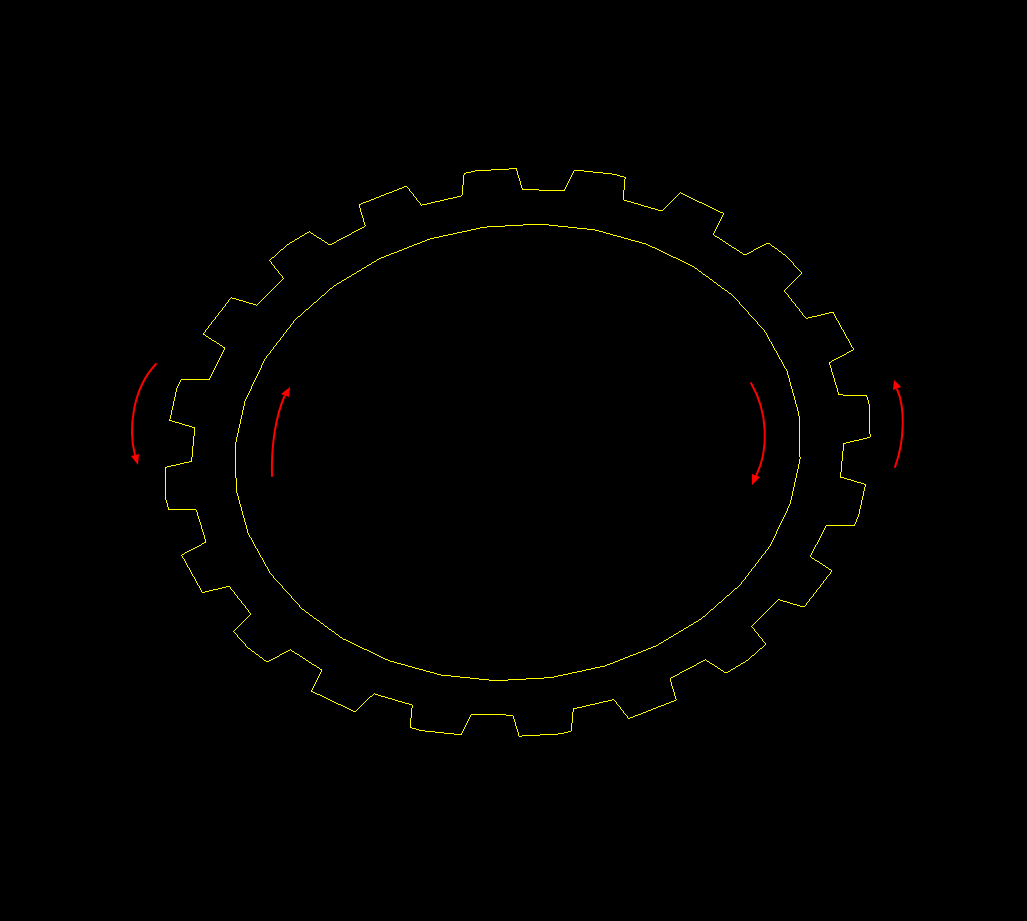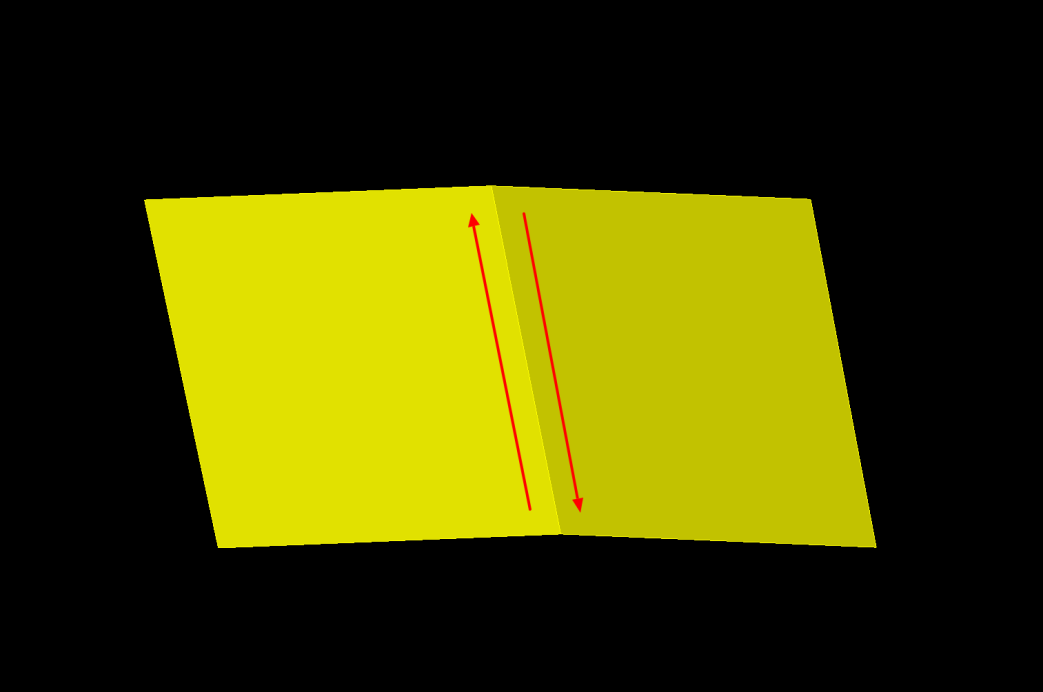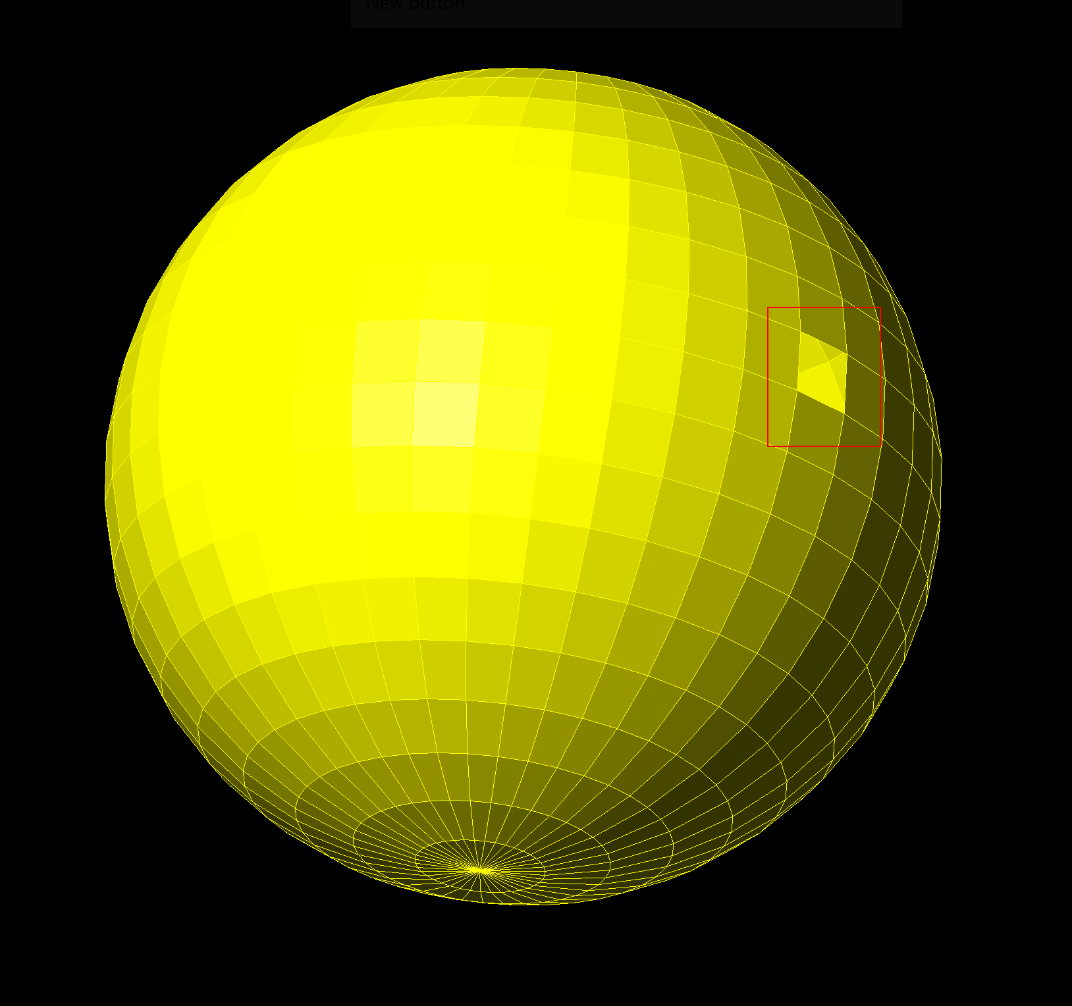# Facet Modeler Specification Overview

## Roman Martens

May 07, 2020

ODA Facet Modeler is a fast modeler that uses boundary representation for solid modeling, also called the B-Rep technique. These are the main data structures used by ODA Facet Modeler:

• Vertex
• Edge
• Edge loop
• Face
• Body

#### Vertex

A vertex is a 3D point.

#### Edge

An edge is an object represented by a pointer to a starting vertex and by pointers to the previous and next edges in the loop. Basically, an edge is a straight segment bounded by two vertices: a start vertex and an end vertex (which is the next edge’s start vertex).

``````Vertex* startVertex = pEdge->vertex();
Vertex* endVertex = pEdge->endVertex();``````

#### Edge Loop

An edge loop is a closed list of non-intersecting edges lying on a 3D plane (consists of at least three edges).

#### Face

A face is a list of non-intersecting edge loops, bounding an area on a 3D plane. A face owns edges through an edge loop.

The first loop is called an outer loop. An outer loop is oriented counter-clockwise when looking at the object in a direction opposite to the plane normal (right-hand rule). All other loops should lie inside the area bounded by the outer loop (if any). They are called inner loops. Inner loops are oriented clockwise.

Here is an example of a face:And here are the directions of outer and inner loops:``````EdgeLoop* pOuterLoop = pFace->loop(0);
EdgeLoop* pInternalLoop = pFace->loop(1);
if (pInternalLoop)
{
// work with internal loop here
}``````

An edge knows which loop and face it belongs to.

``````Face* pLoop = pEdge->loop();
if (pLoop)
{
// work with loop here
}

Face* pFace = pEdge->face();
if (pFace)
{
// work with face here
}``````

Adjacent faces can have a common edge. Topologically, there are two edges called pair-edges. They are formed by the same vertices but in a different order.``````Edge* pPairEdge = pEdge->pair();
if (pPairEdge)
{
// work with pair edge here
}``````

#### Body

A body is a list of connected faces. A body is closed if every edge from one face has a pair-edge from an adjacent face. Otherwise it is considered open.

The example of an open body is shown below (the missing face is in the red highlighted box):Call the Body::isClosed() method to check whether the body is closed.

``bool closed = body.isClosed();``

A body is called consistent if it meets the next conditions:

• Every face has at least one outer loop.
• The outer loop of every face is oriented counter-clockwise.
• The inner loops of every face are oriented clockwise.
• The pair of pair-edges is the original edge.
• Every edge belongs to a face (there are no dangling edges).
• An edge and its pair-edge belong to different faces.

Call the method Body::checkInternalConsistence() to check whether the body is consistent.

``````OdString msgStr;
bool consistency = body.checkInternalConsistence(&msgStr);``````

To learn how to create bodies, see Creating Bodies using the Facet Modeler on the ODA blog.